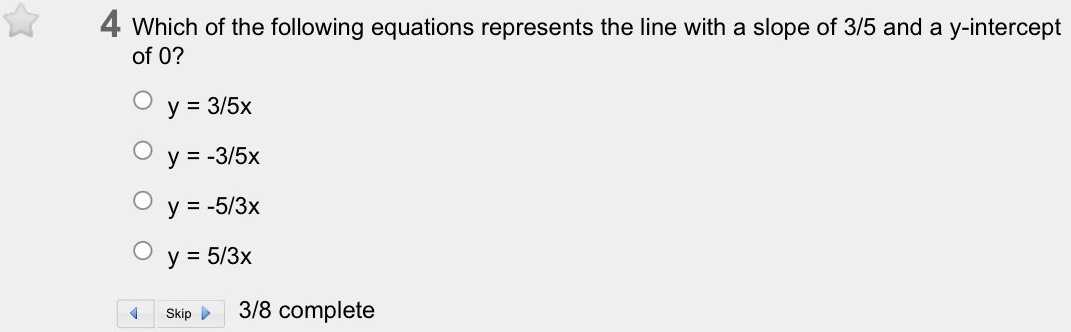### ¿Todavía tienes preguntas de matemáticas?

Pregunte a nuestros tutores expertos
Algebra
Pregunta$$4$$ Which of the following equations represents the line with a slope of $$3 / 5$$ and a $$y$$ -intercept of $$0$$ ?

$$\bigcirc y = 3 / 5 x$$

$$\bigcirc y = - 3 / 5 x$$

$$\bigcirc y = - 5 / 3 x$$

$$\bigcirc y = 5 / 3 x$$

$$y = 3 / 5 x$$